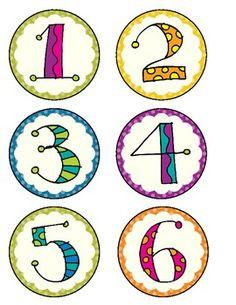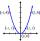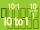# Unknown number

Find an unknown number of which 1/5 is 40 greater than one-tenth of that number.

x =  400

### Step-by-step explanation:Did you find an error or inaccuracy? Feel free to write us. Thank you!

Showing 1 comment:Math student
Triple the number 8 is greater than an unknown numberTips to related online calculators
Need help to calculate sum, simplify or multiply fractions? Try our fraction calculator.
Do you have a linear equation or system of equations and looking for its solution? Or do you have a quadratic equation?

## Related math problems and questions:

• Unknown number 6Determine the unknown number, which is by 1.5 greater than its fourth.
• Unknown numberIf I reduced the sum of the numbers 70 and the unknown number three times, I would get 100. what is the unknown number?
• Unknown number 5I think of an unknown number. If we enlarge it five times, then subtract 3, and the result decreases by 75%, we get one greater than the number. What number am I thinking of?
• Unknown numberFind the unknown number equal to a quarter of a fifth of a number, which is by 152 more than an unknown number.
• FractionsThree-quarters of an unknown number is 4/5. What is 5/6 of this unknown number?
• Unknown number 24f we add 20, we get 50% of its triple. What is this unknown number?
• Unknown numberSamuel wrote unknown number. Then he had add 200000 to the number and the result multiply by three. When it calculated he was surprised, because the result would have received anyway, if write digit to the end of original number. Find unknown number.
• Unknown xIf we add to unknown number 21, then divide by 6 and then subtract 51, we get back an unknown number. What is this unknown number?
• Unknown number 716% of the unknown number is by 21 less than unknown number itself. Determine the natural unknown number.
• UN 1If we add to an unknown number his quarter, we get 210. Identify unknown number.
• NumberDetermine unknown number if you know that difference between five times and triple of number is 42.
• Unknown numbersThe sum of two consecutive natural numbers and their triple is 92. Find these numbers.
• Unknown number 7Calculate unknown number whose 12th power when divided by the 9th power get a number 27 times greater than the unknown number. Determine the unknown number.
• One-thirdA one-third of unknown number is equal to five times as great as the difference of the same unknown number and number 28. Determine the unknown number.
• Unknown mixed numberFind the number that is smaller than 5 5/12 by as much as 2 2/13 is smaller than 6 1/6
• Two fraction equationFind d value of q in the equation 30/16=q/48.
• Two numbersWe have two numbers. Their sum is 140. One-fifth of the first number is equal to half the second number. Determine those unknown numbers.